# Cyclotomic extension

of a fieldAn extensionobtained fromby adjunction of a root of unity (cf. Primitive root). The term is sometimes used for any subextension ofover. An infinite algebraic extension which is the union of finite cyclotomic extensions is also called a cyclotomic extension. Important examples of cyclotomic extensions are provided by the cyclotomic fields (cf. Cyclotomic field), obtained when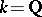is the field of rational numbers.
Letbe of characteristic 0 and let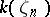be a cyclotomic extension obtained by adjoining a primitive root of unity. Thenis the composite ofand the cyclotomic field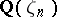. For this reason, many properties of cyclotomic fields carry over to cyclotomic extensions. For example,is an Abelian extension of(this is also true for fields of finite characteristic), the Galois group of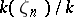is a subgroup of the Galois group of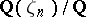; in particular, the order of the former Galois group divides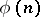, whereis the Euler function.
Ifis an algebraic number field, the only prime divisors that may be ramified inare those dividing, although when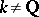a divisor ofdividingmay remain unramified in. The cyclotomic extension of an algebraic number field with Galois groupisomorphic to the additive group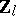of-adic numbers is called the cyclotomic-extension (see , , ). In the case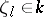this-extension has the form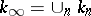, where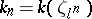.